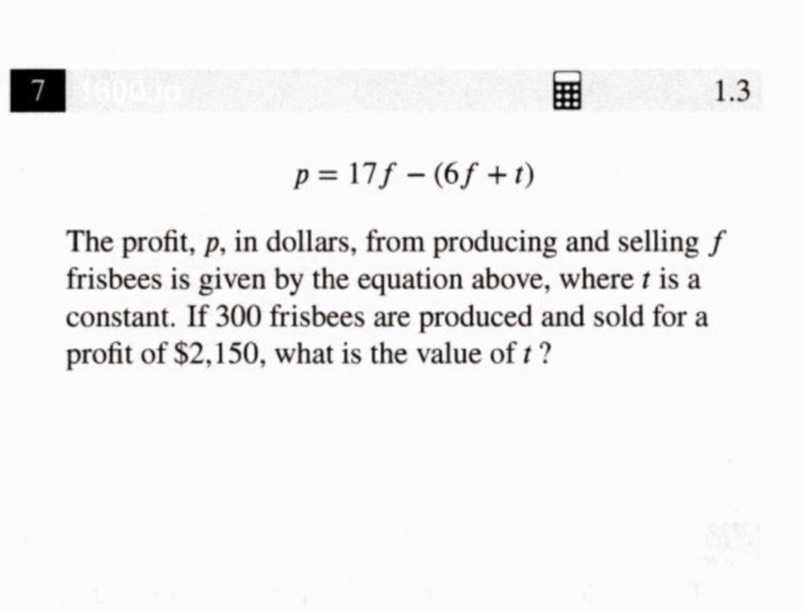### ¿Todavía tienes preguntas de matemáticas?

Pregunte a nuestros tutores expertos
Algebra
Pregunta$$7$$ (1) $$p = 17 f - ( 6 f + t )$$

The profit, $$p$$ , in dollars, from producing and selling $$f$$ frisbees is given by the equation above, where $$t$$ is a constant. If $$300$$ frisbees are produced and sold for a profit of $$\ 2,150$$ , what is the value of $$t$$ ?